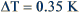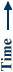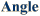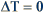# Previous Projects

UNIFORM ROTATING PULSES

The typical bevaior of a spatially-uniform catalyst is spatially synchronized oscillations. Near the boundary between oscillatory and steady-state behavior where the amplitude of oscillation is small, however, a rotating pulse was observed on one occasion. The characteristics of this pulse are quite unlike spatially synchronized oscillations under the same conditions. Typically, the period of oscillation is 120 s and the temperature change is ~8 °C. In this pulse, however, the period of rotation is 15 s and the temperature change was 0.35 °C. This rotating pulse was a transient pattern. After a few thousand rotations (spanning approximately one hour), the catalyst spontaneously underwent a transition to spatially-synchronized, larger amplitude oscillations.

The image below shows a space-time plot of synchronized oscillations on a uniform catalyst. In this plot, the temperature of the catalyst is plotted as a function of angle (horizontal axis) and time (vertical axis). Orange/yellow colors indicate areas where the catalyst is hot and blue represents areas where the catalyst is cool. The slope of the oscillations gives the propagation velocity of the pulse as 0.8 cm/s.S. Y. Yamamoto, C. M. Surko, M. B. Maple, and R. K. Pina,Phys. Rev. Lett. 74, 4071 (1995).

Surko Group: UC San Diego - Department of Physics